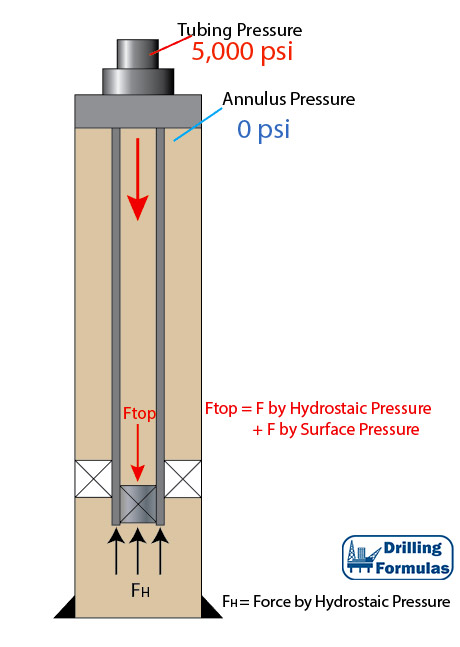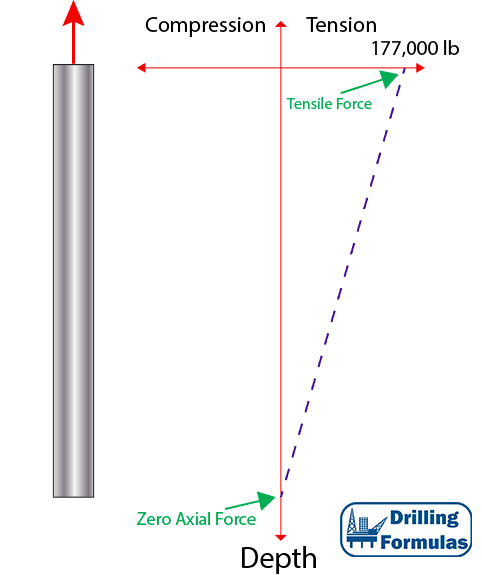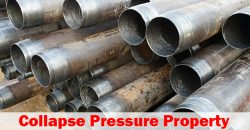## Piston Force on Closed-Ended Tubular (Plugged Tubular)According to the previous post (piston force on open-ended tubular), applied surface pressure will reduce tensile force on surface. In this article, this is an analysis on the piston force on a plugged tubular string and the details are shown below;

Tubing Detail

• 5” Tubing
• ID of tubing = 3.696”
• Packer seal bore OD = 5.25”
• Weight per length = 17.7 lb/ft
• Total Length = 10,000 ft
• Plugged tubing depth = 10,000 ft
• Fluid density = 10 ppg
• Tubing is free to move in the packer
• Applied surface pressure = 5,000 psi

Figure 1 shows the wellbore schematic. Applied pressure (5,000 psi) will cause a piston effect to push the tubing. Therefore, at the bottom of tubing buoyancy and piston force will act in an upwards direction (compression).Figure 1 – Wellbore Schematic

## Piston Force on Open-Ended TubularPiston force is a load caused directly by changes in pressure acting on the exposed cross sectional area of pipe. This results in changing in length of tubular and force acting against tubular. In this article, it generally demonstrates force distribution based on a simple tubular diagram.Tubing Detail

• 5” Tubing
• ID of tubing = 3.696”
• Weight per length = 17.7 lb/ft
• Total Length = 10,000 ft
• Fluid density = 10 ppg
• Tubing is free to move in the packer
• Applied surface pressure = 5,000 psi

## Buoyancy Effect on Weight of Tubular Submersed in FluidWhen a tubular submerses into drilling fluid or completion fluid, it will affect how the force distribution works on the tubular. This article will describe how buoyancy will affect tubular weight and a location of neutral axial load (zero axial load).

Weight of Tubing in Air

When tubing is hung in the air, the string weight is equal to linear weight per foot multiplied by the total length of the string. The maximum tensile load is on surface and the zero axial load point is at the bottom.

Tubing Detail

• 5” Tubing
• Weight per length = 17.7 lb/ft
• Total Length = 10,000 ft

Total weight = 17.7 x 10,000 = 177,000 lb

Weight at the bottom of tubing is 0 and weight on top is 177,000 lb in tensile.

Figure 1 demonstrates force distribution of tubing hanging in air.Figure 1 – Weight of Tubing in Air

Weight of Tubing When Submersed in Fluid Continue reading

## Design Factor for Tubular DesignTubular must be properly deigned to cover all anticipated load cases during the life of the well.  Engineers must select the appropriate tubular grade and weight, which will withstand the loads and be economic for the project. High grade to tubular can lead to excessive cost, which may not be economic viable. However, if the selected tubular is very close to the anticipated load, it might not be safe to operate the well. Therefore, engineers must fully understand the concept about design factors in tubular design.Continue reading

## Collapse Pressure Property for Oilfield TubularCollapse happens when differential between external and internal pressure exceeds a collapse rating pressure of material. This situation can happen due to several cases, such as pressure testing in annulus, trapped pressure in the annulus or well fully evacuated with gas, etc.Figure 1 – Collapse Pressure Diagram

Collapse pressure equations come from experiments from test specimens and the full details can be found in API Bulleting 5C3, Formulas and Calculations for Casing, Tubing, Drillpipe, and Line Pipe Properties. From the experimental results, there are 4 collapse regimes based on Diameter of pipe/Wall thickness (D/t) and yield strength of material which are yield strength collapse, plastic collapse, transitional collapse and elastic collapse. Continue reading